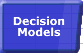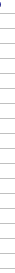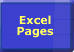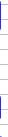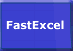# Volatile Excel FunctionsSmart Recalculation Evaluation Circumstances Calculation Process Dependency Trees Controlling Calculation "CALCULATE" in Status Bar Calculation Methods Calculating from VBA Volatile Functions & Actions User-Defined Functions Repetitive Calculation Version Timing Comparison## Volatile Functions.

A Volatile Function is one that causes recalculation of the formula in the cell where it resides every time Excel recalculates.
This occurs regardless of whether the precedent data and formulas on which the formula depends have changed, or whether the formula also contains non-volatile functions.

Avoid volatile functions wherever possible.
You can make a User-Defined Function volatile by including Application.Volatile in the function code.
You can even create conditionally volatile UDFs using Application.Volatile=False and Application.Volatile=True.

XLL UDFs that are registered as Macro type functions (#) and take type R or U arguments are treated as volatile by default.

FastExcel measures Workbook Volatility by comparing the time for a Recalculation to the time for a Full calculation, and allows you to measure worksheet volatility by comparing the sheet recalculate time with the sheet full calculate time.

### Excels Volatile Functions.

Some of Excels functions are obviously volatile: RAND(), NOW(), TODAY()

Others are less obviously volatile: OFFSET(), CELL(), INDIRECT(), INFO(), RANDBETWEEN(), RANDARRAY()

Some are volatile in some versions of Excel but not in others: INDEX()became non-volatile in Excel 97.

A number of functions that are documented by Microsoft as volatile do not actually seem to be volatile when tested:

INDEX(), ROWS(), COLUMNS(), AREAS()

and CELL("Filename") IS volatile although a MSKB article says its not.

One particular syntax of SUMIF is volatile in Excel 2002 and subsequent versions. This occurs when the size of the first range argument is not the same as the second (sum_range) argument.

For example =SUMIF(A1:A4,">0",B1) is volatile whereas =SUMIF(A1:A4,">0",B1:B4) is not volatile. Both of these formulae will reference cells B1:B4.

Presumably this volatile behaviour was added to give correct results when B2:B4 were changed. Thanks to Luke Wisbey for letting me know about this behaviour.

Using a volatile function in a formula will flag the cell containing the formula as volatile, even if the volatile function never gets executed:

=IF(1<2,99,NOW()) will always return 99 and the volatile NOW() function will never be called, but the cell containing the IF formula will be treated as volatile, (thanks to Stephen Bullen for pointing this out).
If cell A1 contains =NOW() then =IF(1<2,99,A1) will always return 99, but the cell containing the IF formula will NOT be treated as volatile.

You can download volatileFuncs.zip for a test workbook that you can use to test if a function or formula is volatile.

### Dependents of Volatile functions.

Direct dependents of volatile functions are always recalculated:
If A1 contains =NOW() and A2 contains =A1 and A3 contains =A2 then both A2 and A3 will be recalculated at each recalculation.

Indirect dependents of volatile functions are not always recalculated:
If A1 contains =NOW(), and A2:A5 contain the numbers 2 to 5 then

• =INDEX(A1:A5,1,1) is directly dependent on volatile cell A1 and will always be recalculated.
• =INDEX(A1:A5,3,1) is only indirectly dependent on volatile cell A1 and will NOT always be recalculated, but it will be recalculated once if for example cell A5 is changed even though the answer will not change.

### IF(), CHOOSE(), AND(), OR()

The IF and CHOOSE functions only execute ONE of the available choices. This is called short-circuiting:

If you create UDFs One, Two and Three each of which contain a debug.print or MsgBox statement so that you can see what is being executed then:

• =IF(True,One(),Two()) will only execute the One() udf, and not the Two() udf.
• =CHOOSE(2,One(),Two(),Three()) will only execute the Two() udf and not the One() or Three() udfs.

The AND and OR functions always execute ALL the available choices:

If the One(), Two() and Three() UDFs return 1,2 and 3 respectively then:

• =AND(One()=2,Two()=2,Three()=3) will always execute the Two() and Three() UDFs even though One()=2 will always be false.
• =OR(One()=1,Two()=1,Three()=1) will always execute the Two() and Three() UDFs even though One()=1 will always be true.

### Volatile only at Workbook Open

References to UDFs where the UDF itself is located in an XLA or external workbook are flagged as dirty when the workbook containing the reference is opened: if calculation is Automatic they will be recalculated at workbook open, if calculation is Manual they will be recalculated at the next recalculation.

Using INDEX as the second part of a range reference, for example A\$2:INDEX(A\$2:A\$8,7,), will also cause the reference to be flagged as dirty when the workbook is opened. (Thanks to Bill Wood for spotting this).

Because a cell has been flagged as dirty the workbook will also be flagged as Unsaved so you may get an unexpected prompt to Save the workbook when closing it.

### Conditional Formats are volatile.

Because conditional formats need to be evaluated at each calculation any formulae used in a conditional format is effectively volatile. Actually conditional formats seem to be super-volatile: they are evaluated each time the cell that contains them is repainted on the screen, even in Manual calculation mode, although VBA functions used in conditional formats will not trigger breakpoints when executed by the repaint.

### Volatile Actions: Actions that trigger calculation

#### Autofilter

Selecting any filtering criteria when using Autofilter will flag ALL the formulae in the autofilter range as uncalculated, even if none of their precedents have changed and even if you select exactly the same filter criteria as before. This can cause Autofilter calculation to be extremely slow.

#### Clicking Row or Column Divider

If calculation is set to Automatic clicking or doubleclicking a row or column divider will trigger a recalculation. But manually changing the height or width of a column or row will NOT trigger a recalculation.

In Manual mode these actions do not flag the workbook as requiring calculation.

#### Inserting or Deleteing Rows, Columns or Cells, or Moving Cells

Insert or Delete Rows or Columns or Cells, or moving Cells (using Drag/Drop or Cut/Paste) anywhere on a Sheet, even to the right or below the Used Range.
AND 
The Worksheet contains cells which:

EITHER -
Are Named
OR -
Contain Formulae with Names referring to this Worksheet or other Worksheets
OR 
Contain Formulae that Refer to other Worksheets
OR 
Contain Formulae that are referred to by other Worksheets

Then these cells become flagged as uncalculated.

#### Adding, Changing or Deleting Defined Names

Any action taken to add, delete, change or alter a defined name or its refersto property will trigger a recalculation.

#### Renaming Worksheets and Changing Worksheet Position

Changing the name of a worksheet or moving it will trigger a recalculation in Automatic mode.
In Manual calculation mode, the statusbar will show "Calculate" ONLY if any formulae on any other sheet refer to the sheet with the changed name/position.

#### Deleteing Worksheets, but not Adding Worksheets

In Automatic mode deleting a worksheet will trigger a recalculation, but adding a worksheet will not.

#### Hiding or Unhiding Rows in Excel 2003

In Excel 2003 hiding or unhiding rows will flag the selected rows as uncalculated, even if no rows were actually hidden or unhidden. If calculation is automatic this will trigger a recalculation. This behaviour is a change from previous versions. Hiding or unhiding columns does NOT flag the column as uncalculated.

Probably the reason for the change is that the SUBTOTAL function in Excel 2003 has an option to include or ignore hidden rows, so Excel needs to be able to trigger a dependency recalculation by dirtying the cells when a row is hidden or unhidden.

#### Opening a .CSV file

Whenever you open a .CSV file, either through VBA or File-->Open, a recalculation of all open workbooks will be triggered, even if calculation is in Manual. Switching off .EnableEvents does not stop this recalculation. The only way I have found to prevent this recalculation from happening is to use VBA to switch Worksheet.EnableCalculation to False for each worksheet that you do NOT want to be calculated.

#### Goal Seek

Each iteration of Goal Seek triggers a recalculation.

See evaluation circumstances for the circumstances that will cause a formula to be evaluated.© 2001-2020 Decision Models Legal Disclaimer Privacy Statement